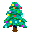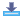www.nikithaonline.comReformers & Politicians | Sports Personalities | Writers & Entertainers | Maximise your Brain Power | General Knowledge | Cartoon Characters | Moral Stories | How? What? Why? When? | Fun with MathematicsStory of Christmas | Dolls | Question Papers | Ramadan Mubarak | Deepavali | Back to HomeScience Computer GK & Reasoning English Grammar MATHS 3rd Grade 4th Grade 8th Grade - 1st Terminal exam 12th Grade - 1st Terminal exam SOCIAL SCIENCE

# Maths - 12th Grade - 1st Terminal exam

1. What is the difference between empty relation and universal relation

2. If A is an invertible matrix of order two, then what is the value of det(A-1)

3. Find the domain of the functions in –1 (2x + 1)/3

4. For a system of Equations, if IAI = 0, where A is a square matrix. How will you examine the consistency of the system of equations

5. If a matrix has 8 elements, what are the possible orders it can have?

6. State chain rule

7. List the relation in the set N given by R= {(a,b) : a=b-2, b>6}

8. Find the value of tan-1 square root of 3 sec-1(-2)

9. If f(x) = x , then what is f(0)

10. If f: R ---à R is defined by f(x) = x/(x2 + 1). Find f (f(2)).

11. Let f,g be the functions f = {(1,5), (2,6), (3,4)}, g= {(4,7), (5,8), (6,9)}. What is the range of fand g.

12. when do we say that a function is invertible . let f:x --ày be an invertible function show that f has unique inverse.

13. i. Find dy/dx, if yx + xy + xx = ab
ii. State and Verify Rolle’s theorem for the function f(x) = x2 + x –6 in the interval [3-2]

14. The sum of three numbers is 6. If we multiply third number with by 3 and add the second number to it we get 11. By adding first and third numbers, we get double of the second number. Represent it algebraically and find the numbers using matrix method.

15. Find the principal values of
i. cos-1 (-square root of 3)/2
ii. cos–1 (-1)Click here to download Question Paper

 @import url(http://www.google.com/cse/api/branding.css);Custom Search